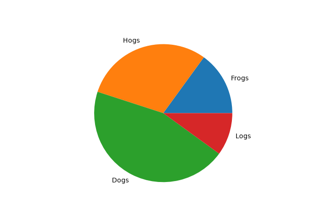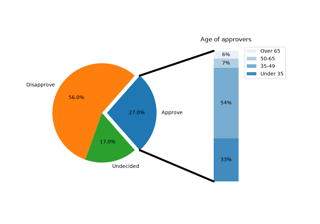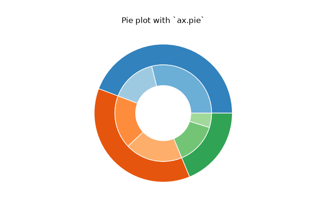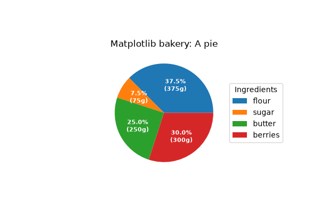# matplotlib.pyplot.pie#

matplotlib.pyplot.pie(x, explode=None, labels=None, colors=None, autopct=None, pctdistance=0.6, shadow=False, labeldistance=1.1, startangle=0, radius=1, counterclock=True, wedgeprops=None, textprops=None, center=(0, 0), frame=False, rotatelabels=False, *, normalize=True, hatch=None, data=None)[source]#

Plot a pie chart.

Make a pie chart of array x. The fractional area of each wedge is given by `x/sum(x)`.

The wedges are plotted counterclockwise, by default starting from the x-axis.

Parameters:
x1D array-like

The wedge sizes.

explodearray-like, default: None

If not None, is a `len(x)` array which specifies the fraction of the radius with which to offset each wedge.

labelslist, default: None

A sequence of strings providing the labels for each wedge

colorscolor or array-like of color, default: None

A sequence of colors through which the pie chart will cycle. If None, will use the colors in the currently active cycle.

hatchstr or list, default: None

Hatching pattern applied to all pie wedges or sequence of patterns through which the chart will cycle. For a list of valid patterns, see Hatch style reference.

New in version 3.7.

autopctNone or str or callable, default: None

If not None, autopct is a string or function used to label the wedges with their numeric value. The label will be placed inside the wedge. If autopct is a format string, the label will be `fmt % pct`. If autopct is a function, then it will be called.

pctdistancefloat, default: 0.6

The relative distance along the radius at which the text generated by autopct is drawn. To draw the text outside the pie, set pctdistance > 1. This parameter is ignored if autopct is `None`.

labeldistancefloat or None, default: 1.1

The relative distance along the radius at which the labels are drawn. To draw the labels inside the pie, set labeldistance < 1. If set to `None`, labels are not drawn but are still stored for use in `legend`.

If bool, whether to draw a shadow beneath the pie. If dict, draw a shadow passing the properties in the dict to `Shadow`.

New in version 3.8: shadow can be a dict.

startanglefloat, default: 0 degrees

The angle by which the start of the pie is rotated, counterclockwise from the x-axis.

counterclockbool, default: True

Specify fractions direction, clockwise or counterclockwise.

wedgepropsdict, default: None

Dict of arguments passed to each `patches.Wedge` of the pie. For example, `wedgeprops = {'linewidth': 3}` sets the width of the wedge border lines equal to 3. By default, `clip_on=False`. When there is a conflict between these properties and other keywords, properties passed to wedgeprops take precedence.

textpropsdict, default: None

Dict of arguments to pass to the text objects.

center(float, float), default: (0, 0)

The coordinates of the center of the chart.

framebool, default: False

Plot Axes frame with the chart if true.

rotatelabelsbool, default: False

Rotate each label to the angle of the corresponding slice if true.

normalizebool, default: True

When True, always make a full pie by normalizing x so that `sum(x) == 1`. False makes a partial pie if `sum(x) <= 1` and raises a `ValueError` for `sum(x) > 1`.

dataindexable object, optional

If given, the following parameters also accept a string `s`, which is interpreted as `data[s]` (unless this raises an exception):

x, explode, labels, colors

Returns:
patcheslist

A sequence of `matplotlib.patches.Wedge` instances

textslist

A list of the label `Text` instances.

autotextslist

A list of `Text` instances for the numeric labels. This will only be returned if the parameter autopct is not None.

Notes

The pie chart will probably look best if the figure and Axes are square, or the Axes aspect is equal. This method sets the aspect ratio of the axis to "equal". The Axes aspect ratio can be controlled with `Axes.set_aspect`.

## Examples using `matplotlib.pyplot.pie`#Pie charts

Pie chartsBar of pie

Bar of pieNested pie charts

Nested pie chartsLabeling a pie and a donut

Labeling a pie and a donut